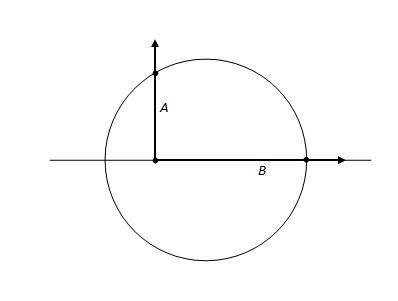# Triangle Optimization problem

## Homework Statement

A right angle is moved along the diameter of a circle of radius a (see diagram). What is the greatest possible length (A+B) intercepted on it by the circle.## Homework Equations

so, the pythagorean theorem might be useful
diameter = 2a

## The Attempt at a Solution

i have to maximise A+B, but i don't exactly have an equation to do that. maybe maximising A2+B2 would work, but that still leaves me with too many variables. i don't know how to relate anything from the circle to the right angle besides the obvious diameter. i know that 0<A<a and 0<B<2a, but this once again gets me nowhere. no idea where to start, please help!

hi ptolema!hint: draw the line from A to the centre of the circle, and call the angle there θ.